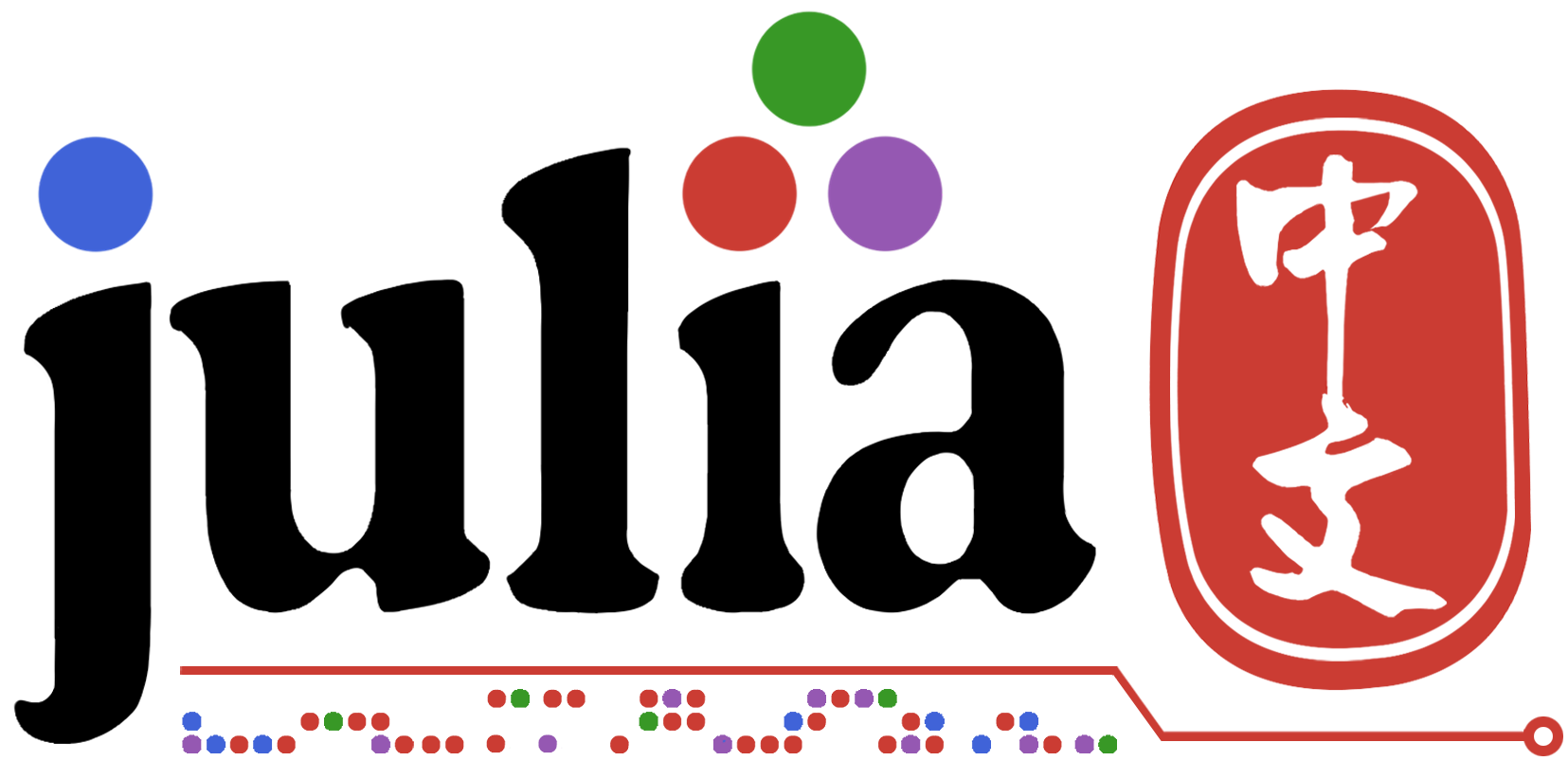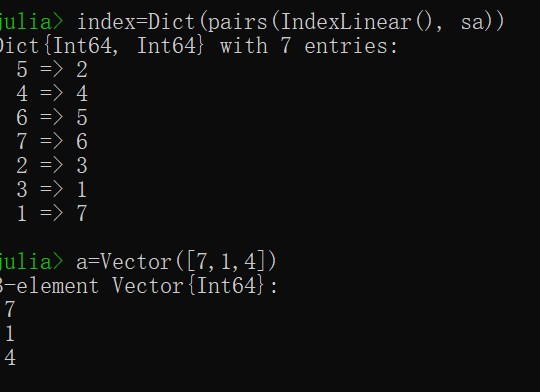# julia如何把字典与向量取交集？

a是一个3个元素的向量，想让他与index这个字典中的value值取交集，返回一个新的3元素字典，应该怎么弄呢?有现成函数么？小白一个``````Dict( i => index[i] for i in a)
``````

``````index = Dict(1=>2, 2=>2, 3=>2）
``````

``````index = Dict(1=>2, 2=>2, 3=>2, 4=>3)
a = 
``````

``````Dict( x=> y for (x,y) in index if  y in a)
``````
1 个赞

``````
julia> index = Dict(5=>2, 4=>4, 6=>5, 7=>6, 2=>3, 3=>1, 1=>7);

julia> a = [7, 1, 4]
3-element Vector{Int64}:
7
1
4

julia> Dict(k=>v for (k,v) in index if v in a)
Dict{Int64, Int64} with 3 entries:
4 => 4
3 => 1
1 => 7
``````